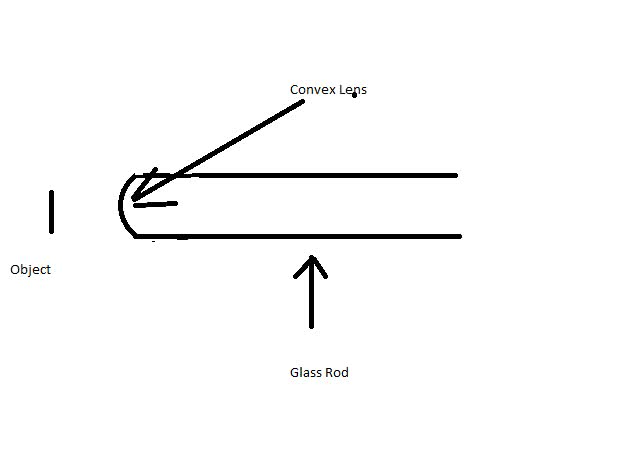1
0
watching
133
views14 Nov 2019

# I'm havingahard time getting the answer to this question. I am usingSnell'slaw of refraction such that: n1/p +n2/q =(n2-n1)/R Where R=12cm n1=1.00 n2=1.60 p=either20cm,8cm, 4cm, 2cm Here isthequestion: One end ofalong glass rod (n = 1.60) has the shape of a convexsurfaceof radius 12.0 cm. An object is positioned in air along theaxis ofthe rod in front of the convex surface. Find the imageposition thatcorresponds to each of the following objectpositions. (a)20.0cm(b) 8.00 cm(c) 4.00 cm(d) 2.00 cmIs this the correct diagram for the question?